Errors in the Second Edition of
Handbook of Differential Equations

LAST UPDATED: April 21, 1998

NOTE THAT THERE MAY EXIST ADDITIONAL ERRORS IN THIS EDITION. PLEASE LOOK AT THE LIST OF ERRATA FOR LATER EDITIONS.

(Great appreciation is extended to Alain Moussiaux for identifying most of the errors listed below.)

1.
On page 13, the definition of the Wronskian contains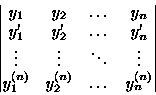This is incorrect, it should have been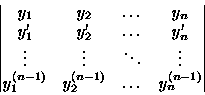2.
On page 37, first line after equation (6.12), we now have
we find that B2-4AC=) and
This is incorrect, it should have been
we find that B2-4AC=0 and

3.
On page 41, line 2 of Special Case 2, the equation beginning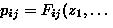'' should have been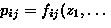''.

4.
On page 48, just above the displayed equation, we now have
If we form the equations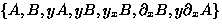, then
This is incorrect, it should be (note the last element in the list)
If we form the equations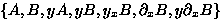, then

5.
On page 55, Note number 1, repeated a'' should be removed.

6.
On page 80, first displayed equation, we now have a cl '' appearing, this should have been cy ''.

7.
On page 81, first theorem, line 3, we now have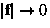'' appearing, this should have been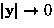''.

8.
On page 82
(a)
Case (A), line 3, we now have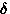exists'' appearing, this should have been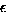exists''.

(b)
Case (C), line 2, we now have hatb '' appearing, this should have been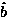''.

(c)
Case (E), line 2, we now have hatb '' appearing, this should have been''.

9.
On page 111, reference 3, year is now 190'', it should have been 1990''.

10.
On page 124
(a)
First displayed equation, we now have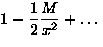'', this should have been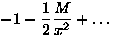''.

(b)
Equation (31.5), we now have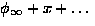'', this should have been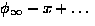''.

11.
On page 126, line -5 , delete the word name''.

12.
On page 127, last line before Transformation 4 is the expression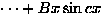'', this should have been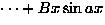''.

13.
On page 129, line 2, the word dependent'' should have been independent''.

14.
On page 145, line -6 , the expression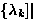'' should have been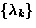''.

15.
On page 157, first displayed equation, the z '' in the numerator on the right hand side should have been a x ''.

16.
On page 169, first displayed equation, the a '' in the second equation should not be present.

17.
On page 186, third word is geberal'', it should be general''.

18.
On page 187
(a)
line -3 , the last term in the equation is (-1)0[an(x)]w '', which is incorrect. It should have been (-1)0[an(x)w] ''.

(b)
line -1 , the first summation is given as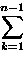'', which is incorrect. It should have been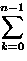''.

19.
On page 194, part (C), the final equation is listed as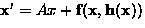''. This should have been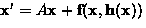''.

20.
On page 195, first displayed equation is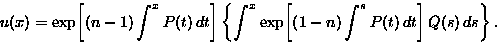This is incorrect, it should have been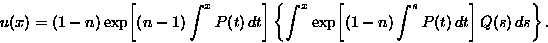21.
On page 224, line 3, to be same'' should have been to be the same''.

22.
On page 228, equation (54.19) and the last displayed equation on the page, the summations are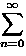'', which are incorrect. They should have been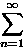''.

23.
On page 234, equation (56.2) is given as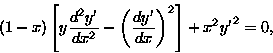This is incorrect, it should have been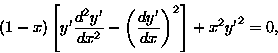24.
On page 244, first displayed equation is now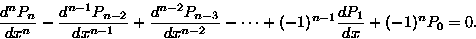This is incorrect, it should have been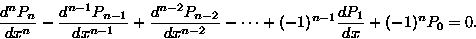25.
On page 249, second to last displayed equation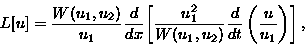This is incorrect, it should have been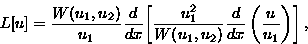26.
On page 250, note number 4, we now have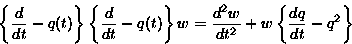This is incorrect, it should have been: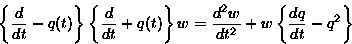27.
On page 260, equation (65.6), we presently have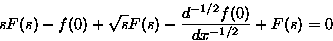This is incorrect, it should have been: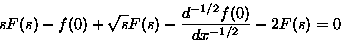28.
On page 274, line -6 , the (68.11)'' should have been (68.8)''.

29.
On page 287, equation (72.18), the middle expression is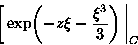This is incorrect, it should have been: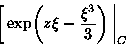30.
On page 305, second to last line on the page, the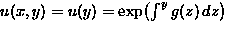'' should have been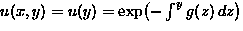''.

31.
On page 307, note number 3, the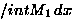)'' should have been)''.

32.
On page 327, equation (80.4) is given as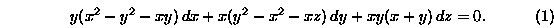This is incorrect, it should have been (note one x should have been a z )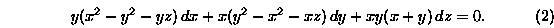33.
On page 331

• The second to last displayed equation on the page is given as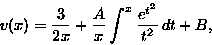This is incorrect, the equation should have been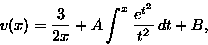• The last displayed equation on the page is given as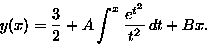This is incorrect, the equation should have been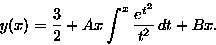34.
On page 333, equation (82.2) is given as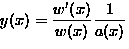. This is incorrect, it should have been (note the minus sign)35.
On page 334, equation (82.6) is given as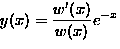. This is incorrect, it should have been (note the minus sign)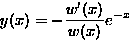36.
On page 339
• equation 84.2 is presently: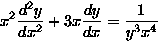.This is incorrect, it should have been: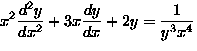.
• last line, the (84.)'' should have been (84.1)''.

37.
On page 358, second displayed equation, second equation is given as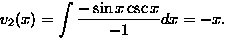''. This is incorrect, it should have been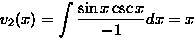.

38.
On page 372:

• Equation (94.17) is given as: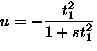This is incorrect, it should have been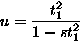.
• Equations (94.18) is given as: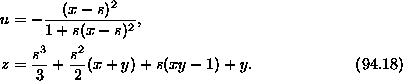This is incorrect, it should have been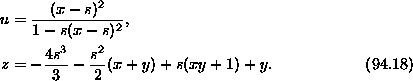39.
On page 385:

• The matrices listed as: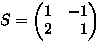and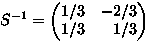are incorrect, they should have been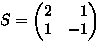and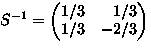.
• Equation (98.4) is listed as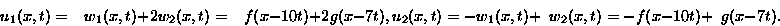This is incorrect, it should have been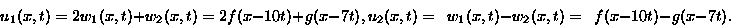40.
On page 386:

• The first displayed equation is: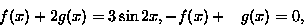This is incorrect, it should have been: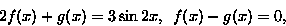• The second displayed equation is: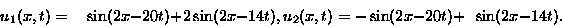This is incorrect, it should have been: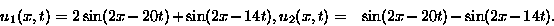41.
On page 392, first line contains were quasilinear''. This is incorrect, it should have been were linear''.

42.
On page 579, the 7th displayed equation is now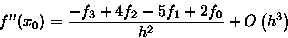the quantity h3 is in error, it should be h2 . That is, the formula should read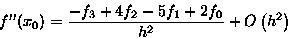43.
On page 581, the first two displayed equations are: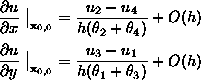and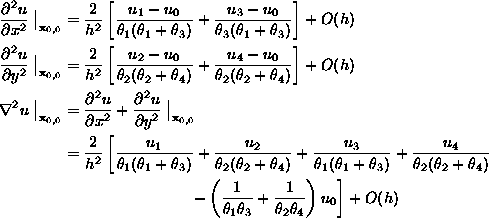They are incorrect, they should have been (note the interchange of x and y in the first two equations, and the three needed modifications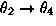,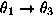, and):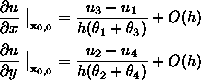and44.
On page 645, equation (166.2), the terms ux and uy should be replaced by vx and vy .